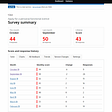# In Wolfram Mathematica, you can have two pure functions on one line

Short post, just wanted to share a neat little discovery I made earlier today. For those of you with lots of experience with Wolfram Mathematica, this might be old hat.

But for those of you who sometimes play around with functional programming with Wolfram Alpha or Scastie or whatever else you use to play around with functional programming, this might be a revelation.

In Wolfram Mathematica, there’s this concept called “pure functions.” With some caveats, you can use pure functions in Wolfram Alpha. In fact, go to Wolfram Alpha right now and type this in:

`Select[Prime[Range], PrimeQ[3# + 4] &]`

This is probably what you would type in if you’re curious about which prime numbers p also give primes for 3p + 4. For example, 5 is such a prime, since 3 × 5 + 4 = 19. By contrast, we see that 7 is not such a prime, since 3 × 7 + 4 = 25 = 5².

Wolfram Alpha gives the correct response to the Wolfram Mathematica statement above: 3, 5, 11, 13, 19, and so on and so forth to 541, which just happens to be the 100th prime number.

So `Range` just gives us the integers 1 to 100, and `Prime[Range]` gives us the primes indexed by the integers 1 to 100, that is, 2, 3, 5, 7, 11, 13, 17, 19, and so on to 541.

Then `Select` tells Mathematica that we want it to select from that list the elements that return `True` for the given Boolean, in this case `PrimeQ[3# + 4] &`.

The `#` “pulls in” a number from the list, multiplies it by 3 and adds 4 (you can write `3 * # + 4` if you prefer). To let Mathematica know we’re invoking a pure function, we need to put `&` before `Select`’s closing brace.

What if you want to iterate 3x + 4 a few times, starting with x = 3 and feeding the result into the next x? In Mathematica, you can use `NestList`. This will also work in Wolfram Alpha:

`NestList[3# + 4 &, 3, 20]`

The `#` here stands first for the initial x, and afterwards for the previous 3x + 4 that becomes the new x. Again we need `&` to let Mathematica know we’re invoking a pure function. Then a comma and our initial argument, 3 in this case. And after that, the 20 means we want it to iterate this twenty times.

The result: 3, 13, 43, 133, 403, 1213, 3643, etc. These numbers get big quick, though we don’t have to worry about overflowing a 32- or 64-bit integer primitive.

As you can see, some of these numbers, like 13 and 43, are prime, others are composite, like 133 = 7 × 19.

This might lead us to wondering: are there prime numbers p such that we can iterate 3x + 4 four times and get only prime numbers?

We could create a Boolean function and use that in a `Select`, something like

`specNumberQ[n_] := (Union[PrimeQ[NestList[3# + 4 &, n, 4]]] == {True});`

This feels inelegant. Even if we don’t save the Mathematica notebook, it seems kind of wasteful to have to name this function we might never use again.

There has got to be a way to use two pure functions in one line (it’s okay if the line wraps for length), one for `NestList`, the other for `Select`.

I won’t bore you with a list of all the wrong things I tried. I do want to make sure you understand that this solution, which is so simple and elegant, wasn’t the first thing I tried, or even the second or third:

`Select[Prime[Range], Union[PrimeQ[NestList[(3# + 4 &), #, 4]]] == {True} &]`

Note that `3# + 4 &` is enclosed in parentheses. That way I tell Mathematica that the scope of the pure function to be iterated is limited to `NestList`’s first argument, enabling Mathematica to understand that the next `#` is meant to stand for a number from `Prime[Range]`.

This won’t work in Wolfram Alpha, though. “Wolfram|Alpha doesn’t understand your query.” Oh well.

Anyway, in Mathematica, this query gives only one result: 3203. We verify that 9613, 28843, 86533 and 259603 are all primes, but 778813 = 7 × 31 × 37 × 97. If we increase 1000 in the `Range` to, say, 10000, we might get more numbers.

More importantly, however, if you ever need to use two pure functions in one line in Mathematica, now you know how: use parentheses.

Actually, as it turns out, at least for this particular example, the parentheses are unnecessary. Just now I verified that

`Select[Prime[Range], Union[PrimeQ[NestList[3# + 4 &, #, 4]]] == {True} &]`

gives the correct result. With the proper placement of the ampersands, Mathematica will correctly understand where the argument for each pure function comes from.

I hope you get some use out of this, or at least think it might be useful to you in the future.

--

--

--

## More from Alonso Del Arte

is a composer and photographer from Detroit, Michigan. He has been working on a Java program to display certain mathematical diagrams.

Love podcasts or audiobooks? Learn on the go with our new app.

## Kafka Operation, The Intelligent Way!## Introduction to Red-Black Trees## Build your own TrumpText in less than an hour## Applying SOLID Principles with C### Weeknotes S01 E14## Collections in Java## 10 Web Development Trends Every CTO Should Expect in 2021## SymfonyCon Lisbon summary## Alonso Del Arte

is a composer and photographer from Detroit, Michigan. He has been working on a Java program to display certain mathematical diagrams.

## Did you know that windows have a built-in Free Malicious scanner?## How to use iMaps like a developer## A Driving Tour of Modern Homes in Los Angeles## “Riding a bike is a unique sensation that no one should miss out on” — Saleta Castro